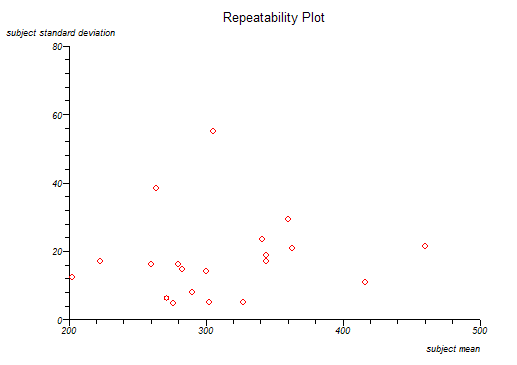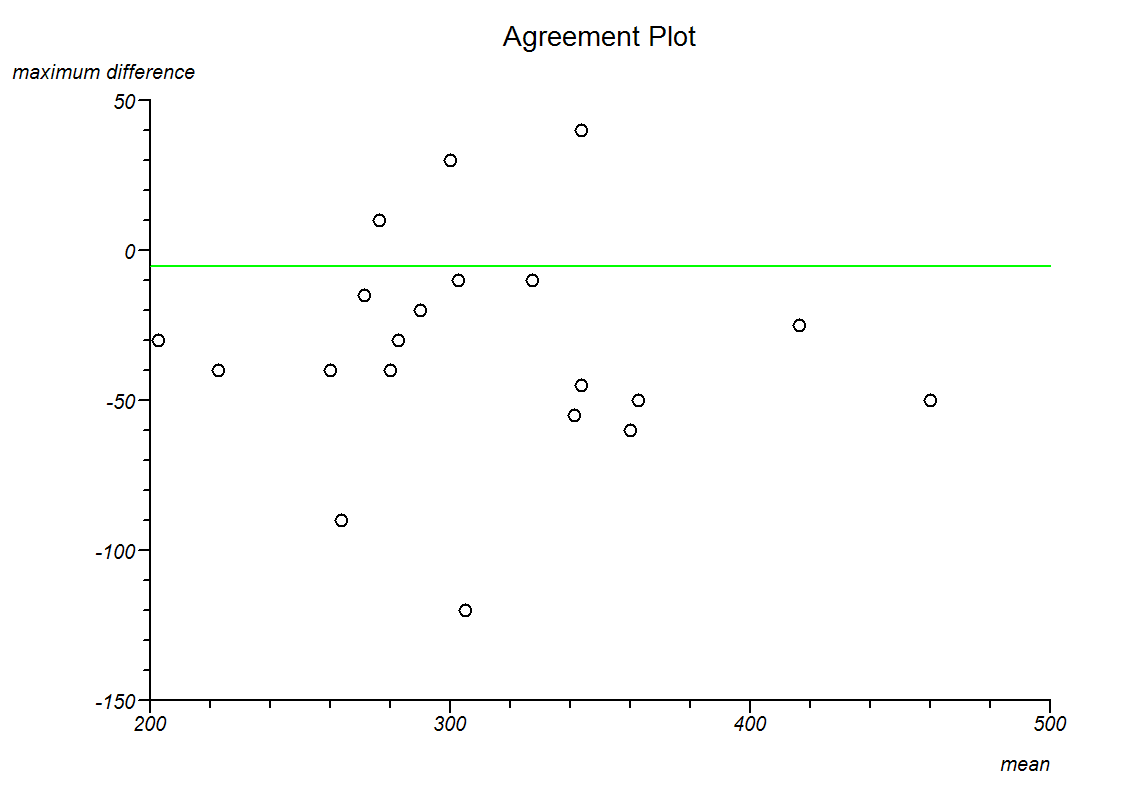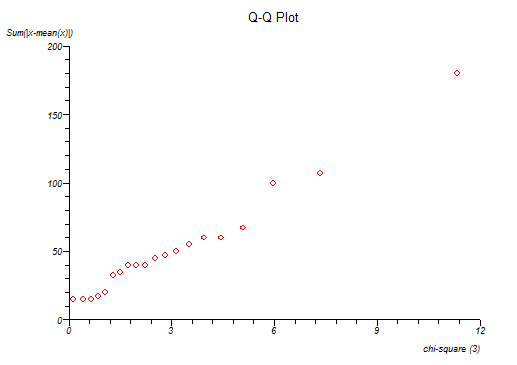# Agreement of Continuous Measurements

Menu location: Analysis_Agreement_Continuous (Intra-class).

The function calculates one way random effects intra-class correlation coefficient, estimated within-subjects standard deviation and a repeatability coefficient (Bland and Altman 1996a and 1996b, McGraw and Wong, 1996).

Intra-class correlation coefficient is calculated as:- where m is the number of observations per subject, SSB is the sum of squared between subjects and SST is the total sum of squares (as per one way ANOVA above).

Within-subjects standard deviation is estimated as the square root of the residual mean square from one way ANOVA.

The repeatability coefficient is calculated as:- where m is the number of observations per subject, z is a quantile from the standard normal distribution (usually taken as the 5% two tailed quantile of 1.96) and ζw is the estimated within-subjects standard deviation (calculated as above).

Intra-subject standard deviation is plotted against intra-subject means and Kendall's rank correlation is used to assess the interdependence of these two variables.

An agreement plot is constructed by plotting the maximum differences from each possible intra-subject contrast against intra-subject means and the overall mean is marked as a line on this plot.

A Q-Q plot is given; here the sum of the difference between intra-subject observations and their means are ordered and plotted against an equal order of chi-square quantiles.

Agreement analysis is best carried out under expert statistical guidance.

Example

Test workbook (Agreement worksheet: 1st, 2nd, 3rd, 4th).

Five peak flow measurements were repeated for twenty children:

 1st 2nd 3rd 4th 190 220 200 200 220 200 240 230 260 260 240 280 210 300 280 265 270 265 280 270 280 280 270 275 260 280 280 300 275 275 275 305 280 290 300 290 320 290 300 290 300 300 310 300 270 250 330 370 320 330 330 330 335 320 335 375 350 320 340 365 360 320 350 345 330 340 380 390 335 385 360 370 400 420 425 420 430 460 480 470

To analyse these data using StatsDirect you must first enter them into a workbook or open the test workbook. Then select Continuous from the Agreement section of the Analysis menu.

Agreement

Variables: 1st, 2nd, 3rd, 4th

Intra-class correlation coefficient (one way random effects) = 0.882276

Estimated within-subjects standard deviation = 21.459749

For within-subjects sd vs. mean, Kendall's tau b = 0.164457 two sided P = .3296

Repeatability (for alpha = 0.05) = 59.482297